Download Presentation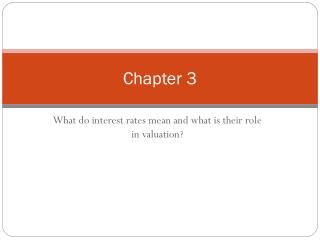Chapter 3

# Chapter 3 - PowerPoint PPT Presentation

Chapter 3. What do interest rates mean and what is their role in valuation?. Present Value Introduction. Different debt instruments have very different streams of cash payments to the holder (known as cash flows ), with very different timing.I am the owner, or an agent authorized to act on behalf of the owner, of the copyrighted work described.
Download Presentation## Chapter 3

An Image/Link below is provided (as is) to download presentation

Download Policy: Content on the Website is provided to you AS IS for your information and personal use and may not be sold / licensed / shared on other websites without getting consent from its author.While downloading, if for some reason you are not able to download a presentation, the publisher may have deleted the file from their server.

- - - - - - - - - - - - - - - - - - - - - - - - - - E N D - - - - - - - - - - - - - - - - - - - - - - - - - -
Presentation Transcript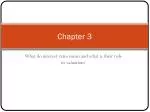### Chapter 3

What do interest rates mean and what is their role in valuation?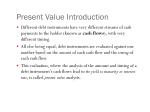Present Value Introduction
• Different debt instruments have very different streams of cash payments to the holder (known as cash flows), with very different timing.
• All else being equal, debt instruments are evaluated against one another based on the amount of each cash flow and the timing of each cash flow.
• This evaluation, where the analysis of the amount and timing of a debt instrument’s cash flows lead to its yield to maturity or interest rate, is called present value analysis.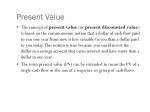Present Value
• The concept of present value (or present discounted value) is based on the commonsense notion that a dollar of cash flow paid to you one year from now is less valuable to you than a dollar paid to you today. This notion is true because you could invest the dollar in a savings account that earns interest and have more than a dollar in one year.
• The term present value (PV) can be extended to mean the PV of a single cash flow or the sum of a sequence or group of cash flows.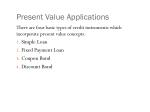Present Value Applications

There are four basic types of credit instruments which incorporate present value concepts:

• Simple Loan
• Fixed Payment Loan
• Coupon Bond
• Discount Bond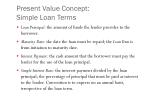Present Value Concept: Simple Loan Terms
• Loan Principal: the amount of funds the lender provides to the borrower.
• Maturity Date: the date the loan must be repaid; the Loan Term is from initiation to maturity date.
• Interest Payment: the cash amount that the borrower must pay the lender for the use of the loan principal.
• Simple Interest Rate: the interest payment divided by the loan principal; the percentage of principal that must be paid as interest to the lender. Convention is to express on an annual basis, irrespective of the loan term.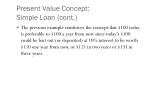Present Value Concept: Simple Loan (cont.)
• The previous example reinforces the concept that \$100 today is preferable to \$100 a year from now since today’s \$100 could be lent out (or deposited) at 10% interest to be worth \$110 one year from now, or \$121 in two years or \$133 in three years.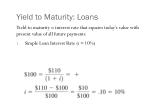Yield to Maturity: Loans

Yield to maturity = interest rate that equates today’s value with present value of all future payments

• Simple Loan Interest Rate (i= 10%)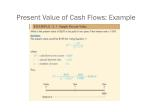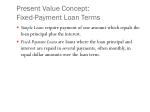Present Value Concept: Fixed-Payment Loan Terms
• Simple Loans require payment of one amount which equals the loan principal plus the interest.
• Fixed-PaymentLoans are loans where the loan principal and interest are repaid in several payments, often monthly, in equal dollar amounts over the loan term.Present Value Concept: Fixed-Payment Loan Terms
• Installment Loans, such as auto loans and home mortgages are frequently of the fixed-payment type.Yield to Maturity: Loans
• Fixed Payment Loan (i= 12%)Yield to Maturity: Bonds
• Coupon Bond (Coupon rate = 10% =C/F)

Consol: Fixed coupon payments of \$C foreverYield to Maturity: Bonds
• One-Year Discount Bond (P= \$900, F= \$1000)Relationship Between Price and Yield to Maturity
• Three interesting facts in Table 3.1
• When bond is at par, yield equals coupon rate
• Price and yield are negatively related
• Yield greater than coupon rate when bond price is below par valueRelationship Between Price and Yield to Maturity
• It’s also straight-forward to show that the value of a bond (price) and yield to maturity (YTM) are negatively related. If i increases, the PV of any given cash flow is lower; hence, the price of the bond must be lower.Current Yield
• Current yield (CY) is just an approximation for YTM—easier to calculate. However, we should be aware of its properties:
• If a bond’s price is near par and has a long maturity, then CY is a good approximation.
• A change in the current yield always signals change in same direction as yield to maturityYield on a Discount Basis
• One-Year Bill (P = \$900, F = \$1000)
• Two Characteristics
• Understates yield to maturity; longer the maturity, greater is understatement
• Change in discount yield always signals change in same direction as yield to maturityGlobal perspective
• In November 1998, rates on Japanese 6-month government bonds were negative! Investors were willing to pay more than they would receive in the future.
• Best explanation is that investors found the convenience of the bills worth something—more convenient than cash. But that can only go so far—the rate was only slightly negative.Distinction Between Real and Nominal Interest Rates
• Real interest rate
• Interest rate that is adjusted for expected changes in the price level

ir = i – pe

• Real interest rate more accurately reflects true cost of borrowing
• When the real rate is low, there are greater incentives to borrow and less to lendDistinction Between Real and Nominal Interest Rates
• Real interest rate

ir = i – pe

We usually refer to this rate as the ex ante real rate of interest because it is adjusted for the expected level of inflation. After the fact, we can calculate the ex post real rate based on the observed level of inflation.Distinction Between Real and Nominal Interest Rates (cont.)
• If i= 5% and pe= 0% then
• If i= 10% and pe= 20% thenU.S. Real and Nominal Interest Rates

Sample of current rates and indexes

http://www.martincapital.com/charts.htmDistinction Between Interest Rates and Returns
• Rate of Return: we can decompose returns into two pieces:Key Facts about the Relationship Between Rates and Returns

Sample of current coupon rates and yields on government bonds

http://www.bloomberg.com/markets/iyc.htmlMaturity and the Volatility of Bond Returns
• Key findings from Table 3.2
• Only bond whose return = yield is one with maturity = holding period
• For bonds with maturity > holding period, i P  implying capital loss
• Longer is maturity, greater is price change associated with interest rate changeMaturity and the Volatility of Bond Returns (cont.)
• Key findings from Table 3.2 (continued)
• Longer is maturity, more return changes with change in interest rate
• Bond with high initial interest rate can still have negative return if iMaturity and the Volatility of Bond Returns (cont.)
• Conclusion from Table 3.2 analysis
• Prices and returns more volatile for long-term bonds because have higher interest-rate risk
• No interest-rate risk for any bond whose maturity equals holding periodReinvestment Risk
• Occurs if hold series of short bonds over long holding period
• i at which reinvest uncertain
• Gain from i, lose when i Formula for Duration
• Key facts about duration
• All else equal, when the maturity of a bond lengthens, the duration rises as well
• All else equal, when interest rates rise, the duration of a coupon bond fallFormula for Duration
• The higher is the coupon rate on the bond, the shorter is the duration of the bond
• Duration is additive: the duration of a portfolio of securities is the weighted-average of the durations of the individual securities, with the weights equaling the proportion of the portfolio invested in eachDuration and Interest-Rate Risk
• i 10% to 11%:
• Table 3.4, 10% coupon bondDuration and Interest-Rate Risk (cont.)
• i 10% to 11%:
• 20% coupon bond, DUR = 5.72 yearsDuration and Interest-Rate Risk (cont.)
• The greater is the duration of a security, the greater is the percentage change in the market value of the security for a given change in interest rates
• Therefore, the greater is the duration of a security, the greater is its interest-rate risk